# JP5626435B2 - Direct AC power converter - Google Patents

## Info

Publication number
JP5626435B2
JP5626435B2 JP2013199760A JP2013199760A JP5626435B2 JP 5626435 B2 JP5626435 B2 JP 5626435B2 JP 2013199760 A JP2013199760 A JP 2013199760A JP 2013199760 A JP2013199760 A JP 2013199760A JP 5626435 B2 JP5626435 B2 JP 5626435B2
Authority
JP
Japan
Prior art keywords
voltage
duty
capacitor
current
period
Prior art date
Legal status (The legal status is an assumption and is not a legal conclusion. Google has not performed a legal analysis and makes no representation as to the accuracy of the status listed.)
Active
Application number
JP2013199760A
Other languages
Japanese (ja)
Other versions
JP2014082926A (en
Inventor

Original Assignee
ダイキン工業株式会社
Priority date (The priority date is an assumption and is not a legal conclusion. Google has not performed a legal analysis and makes no representation as to the accuracy of the date listed.)
Filing date
Publication date
Priority to JP2012214270 priority Critical
Priority to JP2012214270 priority
Application filed by ダイキン工業株式会社 filed Critical ダイキン工業株式会社
Priority to JP2013199760A priority patent/JP5626435B2/en
Publication of JP2014082926A publication Critical patent/JP2014082926A/en
Application granted granted Critical
Publication of JP5626435B2 publication Critical patent/JP5626435B2/en
Active legal-status Critical Current
Anticipated expiration legal-status Critical

• 239000003990 capacitor Substances 0.000 claims description 92
• 238000007599 discharging Methods 0.000 claims description 13
• 238000006243 chemical reactions Methods 0.000 claims description 2
• 238000000034 methods Methods 0.000 description 18
• 230000001629 suppression Effects 0.000 description 4
• 230000001276 controlling effects Effects 0.000 description 3
• 230000000694 effects Effects 0.000 description 2
• 230000001939 inductive effects Effects 0.000 description 2

## Images

•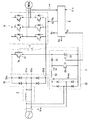••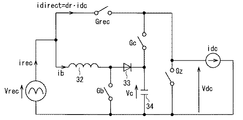•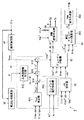••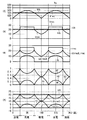•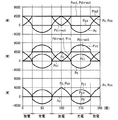•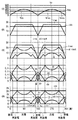•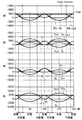•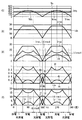•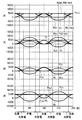•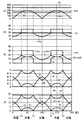•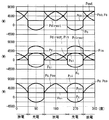•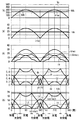•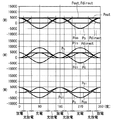•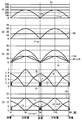•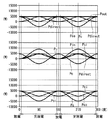## Classifications

• HELECTRICITY
• H02GENERATION; CONVERSION OR DISTRIBUTION OF ELECTRIC POWER
• H02MAPPARATUS FOR CONVERSION BETWEEN AC AND AC, BETWEEN AC AND DC, OR BETWEEN DC AND DC, AND FOR USE WITH MAINS OR SIMILAR POWER SUPPLY SYSTEMS; CONVERSION OF DC OR AC INPUT POWER INTO SURGE OUTPUT POWER; CONTROL OR REGULATION THEREOF
• H02M5/00Conversion of ac power input into ac power output, e.g. for change of voltage, for change of frequency, for change of number of phases
• H02M5/40Conversion of ac power input into ac power output, e.g. for change of voltage, for change of frequency, for change of number of phases with intermediate conversion into dc
• H02M5/42Conversion of ac power input into ac power output, e.g. for change of voltage, for change of frequency, for change of number of phases with intermediate conversion into dc by static converters
• H02M5/44Conversion of ac power input into ac power output, e.g. for change of voltage, for change of frequency, for change of number of phases with intermediate conversion into dc by static converters using discharge tubes or semiconductor devices to convert the intermediate dc into ac
• H02M5/453Conversion of ac power input into ac power output, e.g. for change of voltage, for change of frequency, for change of number of phases with intermediate conversion into dc by static converters using discharge tubes or semiconductor devices to convert the intermediate dc into ac using devices of a triode or transistor type requiring continuous application of a control signal
• H02M5/458Conversion of ac power input into ac power output, e.g. for change of voltage, for change of frequency, for change of number of phases with intermediate conversion into dc by static converters using discharge tubes or semiconductor devices to convert the intermediate dc into ac using devices of a triode or transistor type requiring continuous application of a control signal using semiconductor devices only
• HELECTRICITY
• H02GENERATION; CONVERSION OR DISTRIBUTION OF ELECTRIC POWER
• H02MAPPARATUS FOR CONVERSION BETWEEN AC AND AC, BETWEEN AC AND DC, OR BETWEEN DC AND DC, AND FOR USE WITH MAINS OR SIMILAR POWER SUPPLY SYSTEMS; CONVERSION OF DC OR AC INPUT POWER INTO SURGE OUTPUT POWER; CONTROL OR REGULATION THEREOF
• H02M1/00Details of apparatus for conversion
• H02M1/42Circuits or arrangements for compensating for or adjusting power factor in converters or inverters
• H02M1/4208Arrangements for improving power factor of AC input
• H02M1/4225Arrangements for improving power factor of AC input using a non-isolated boost converter
• HELECTRICITY
• H02GENERATION; CONVERSION OR DISTRIBUTION OF ELECTRIC POWER
• H02MAPPARATUS FOR CONVERSION BETWEEN AC AND AC, BETWEEN AC AND DC, OR BETWEEN DC AND DC, AND FOR USE WITH MAINS OR SIMILAR POWER SUPPLY SYSTEMS; CONVERSION OF DC OR AC INPUT POWER INTO SURGE OUTPUT POWER; CONTROL OR REGULATION THEREOF
• H02M7/00Conversion of ac power input into dc power output; Conversion of dc power input into ac power output
• H02M7/02Conversion of ac power input into dc power output without possibility of reversal
• H02M7/04Conversion of ac power input into dc power output without possibility of reversal by static converters
• H02M7/06Conversion of ac power input into dc power output without possibility of reversal by static converters using discharge tubes without control electrode or semiconductor devices without control electrode
• H02M7/08Conversion of ac power input into dc power output without possibility of reversal by static converters using discharge tubes without control electrode or semiconductor devices without control electrode arranged for operation in parallel
• HELECTRICITY
• H02GENERATION; CONVERSION OR DISTRIBUTION OF ELECTRIC POWER
• H02JCIRCUIT ARRANGEMENTS OR SYSTEMS FOR SUPPLYING OR DISTRIBUTING ELECTRIC POWER; SYSTEMS FOR STORING ELECTRIC ENERGY
• H02J3/00Circuit arrangements for ac mains or ac distribution networks
• H02J3/36Arrangements for transfer of electric power between ac networks via a high-tension dc link
• YGENERAL TAGGING OF NEW TECHNOLOGICAL DEVELOPMENTS; GENERAL TAGGING OF CROSS-SECTIONAL TECHNOLOGIES SPANNING OVER SEVERAL SECTIONS OF THE IPC; TECHNICAL SUBJECTS COVERED BY FORMER USPC CROSS-REFERENCE ART COLLECTIONS [XRACs] AND DIGESTS
• Y02TECHNOLOGIES OR APPLICATIONS FOR MITIGATION OR ADAPTATION AGAINST CLIMATE CHANGE
• Y02BCLIMATE CHANGE MITIGATION TECHNOLOGIES RELATED TO BUILDINGS, e.g. HOUSING, HOUSE APPLIANCES OR RELATED END-USER APPLICATIONS
• Y02B70/00Technologies for an efficient end-user side electric power management and consumption
• Y02B70/10Technologies improving the efficiency by using switched-mode power supplies [SMPS], i.e. efficient power electronics conversion
• Y02B70/12Power factor correction technologies for power supplies
• Y02B70/126Active technologies

## Description

The present invention relates to a direct AC power converter, and more particularly to a configuration including a rectifier circuit, an inverter, and a boost chopper.

Patent Documents 1 and 2 and Non-Patent Document 1 introduce direct AC power converters. In these direct AC power converters, a step-up chopper is used, which receives power from the rectifier circuit and supplies power to the inverter, thereby reducing pulsation of instantaneous power.

JP 2011-193678 A JP 2012-135184 A

Onuma, Ito, "Verification of a single-phase three-phase power converter with an active buffer with a charging circuit" 2010 IEEJ Industrial Application Division Conference 1-124, I-587-590

However, in the techniques introduced in these documents, the period for charging from the rectifier circuit to the capacitor and the period for discharging from the capacitor to the inverter are set alternately every 1/4 of the period of the input AC voltage. It was. For this reason, the magnitude of the output voltage remained at 1 / √2 times the peak value of the input AC voltage.

Further, the operation of the step-up chopper is performed in a so-called discontinuous mode, and the peak current flowing through the inductor included in the step-up chopper tends to increase.

Accordingly, an object of the present invention is to reduce a peak current flowing in an inductor included in a boost chopper. Another object of the present invention is to provide a technique capable of increasing the output voltage from the direct AC power conversion device as compared with the prior art.

The direct AC power converter according to the present invention includes a DC link (7), a first rectifier circuit (5, 51), a boost chopper (3), and a switch (41).

The DC link has a first power supply line (L1) and a second power supply line (L2) to which a potential lower than the potential of the first power supply line is applied.

The first rectifier circuit has a plurality of input terminals for inputting an AC voltage, and a pair of output terminals (51c, 51d) connected to the DC link.

The inverter converts a voltage applied to the DC link into another AC voltage.

The step-up chopper has a capacitor (34) at the output stage.

The switch performs discharge / non-discharge from the capacitor to the DC link.

In the first aspect of the direct AC power converting apparatus according to the present invention, the capacitor is charged in the boost chopper at least in a first period that is a part of a period in which the discharge duty (dc) is greater than zero. Is called.

The discharge duty is a ratio at which the switch is turned on.

A second aspect of the direct AC power converting apparatus according to the present invention is the first aspect, wherein the step-up chopper is in part or all of the first period and the period when the discharge duty is zero. The capacitor is charged in

A third aspect of the direct AC power converting apparatus according to the present invention is any one of the first and second aspects, and in the first period, charging power (Pci) and discharging power ( The capacitor (34) is charged and discharged by Pco), and the first period has a period in which the charging power is larger than the discharging power and a period in which the discharging power is larger than the charging power.

A fourth aspect of the direct AC power converting apparatus according to the present invention is any one of the first to third aspects, wherein the virtual DC link voltage (Vdc) is 1 / √ of the rectified voltage. Greater than twice.

The virtual DC link voltage includes a product (dc · Vc) of the discharge duty (dc) and a voltage (Vc) across the capacitor (34), a rectification duty (dr), and a rectification voltage (Vrec) of the AC voltage. And the product (dr · Vrec).

The rectification duty is a value obtained by subtracting the sum of the discharge duty and the zero voltage duty (dz) from 1.

The zero voltage duty is a time ratio at which the inverter (6) adopts a zero voltage vector regardless of the voltage output from the inverter (6).

A fifth aspect of the direct AC power converting apparatus according to the present invention, in the fourth state like its, the boost chopper (3) to the current flowing (ib) and the inverter from the first rectifier circuit ( 6) a command value (irec *) of the rectified current (irec), which is the sum of the current flowing through (direct), a command value (Vdc *) of the virtual DC link voltage (Vdc), and a voltage across the capacitor ( The discharge duty that minimizes the zero voltage duty (dz) is set based on Vc), the rectified voltage (Vrec), and the current (idc) input to the inverter (6).

A sixth aspect of the direct AC power converting apparatus according to the present invention is the fifth aspect , wherein the virtual DC link voltage (Vdc) is larger than the rectified voltage (Vrec), and the rectified current (irec) ) Is equal to or greater than a predetermined value (dr_max), the commutation duty (dr) takes the predetermined value, and the discharge duty (dc) is a value obtained by subtracting the predetermined value from 1. take.

The predetermined value is obtained by subtracting the both-end voltage (Vc) of the capacitor from the command value (Vdc *) of the virtual DC link voltage (Vdc), and the both-end voltage (Vc) from the rectified voltage (Vrec). It is a value ((Vdc * −Vc) / (Vrec−Vc)) divided by the subtracted value.

A seventh aspect of the direct AC power converting apparatus according to the present invention is any one of the first to sixth aspects, and inputs the rectified voltage (Vrec) to the boost chopper (3). A second rectifier circuit (52) is further provided.

An eighth aspect of the direct AC power converting apparatus according to the present invention is any one of the first to sixth aspects, wherein the pair of output terminals of the first rectifier circuit are connected to the boost chopper. Connected to the input side.

A ninth aspect of the direct AC power converting apparatus according to the present invention is any one of the first aspect and the third to eighth aspects , and the discharge duty (dc) is always positive.

A tenth aspect of the direct AC power converting apparatus according to the present invention is any one of the first to ninth aspects, wherein the direct current AC power converter is connected to the switch (41) in parallel with the DC link (7). It further includes a diode whose forward direction is the direction of charging the capacitor (34).

At least in part of the period when the discharge duty is greater than 0, the capacitor is charged by the boost chopper, so that the average value of the maximum voltage that can be output by the inverter and the period for controlling the inverter switching is AC. It can exceed 1 / √2 times the peak value of the voltage.

Alternatively, even if the average value does not exceed 1 / √2 times the peak value of the AC voltage, the capacitor charging period can be selected to be longer than in the prior art, so that the current flowing in the inductor of the boost chopper can be selected. The peak value can be reduced as compared with the conventional technique.

It is a circuit diagram which illustrates the composition of the direct form AC power converter concerning this embodiment. It is a circuit diagram which illustrates other composition of a direct form exchange power converter concerning this embodiment. It is an equivalent circuit of the direct AC power converter according to this embodiment. It is a block diagram which illustrates the composition of the control part in the direct form AC power converter concerning this embodiment. It is the flowchart which put together the process of calculating | requiring a commutation duty, a zero voltage duty, a discharge duty, and an electric current command value. 10 is a graph showing the waveform of specifications of Comparative Example 1. 10 is a graph showing the waveform of specifications of Comparative Example 1. 3 is a graph showing the waveform of specifications of Example 1. 3 is a graph showing the waveform of specifications of Example 1. 6 is a graph showing the waveform of specifications of Example 2. 6 is a graph showing the waveform of specifications of Example 2. 10 is a graph showing waveforms of specifications of Comparative Example 2. 10 is a graph showing waveforms of specifications of Comparative Example 2. 10 is a graph showing the waveform of specifications of Example 3. 10 is a graph showing the waveform of specifications of Example 3. It is a graph which shows the waveform of the item of Example 4. It is a graph which shows the waveform of the item of Example 4.

FIG. 1 is a circuit diagram illustrating the configuration of a direct AC power converting apparatus according to this embodiment.

The direct AC power converter includes a DC link 7, rectifier circuits 51 and 52, a boost chopper 3, a switch 41, and an inverter 6.

The DC link 7 has power supply lines L1 and L2. A potential lower than the potential of the power supply line L1 is applied to the power supply line L2.

The rectifier circuit 51 has input terminals 51a and 51b for inputting the AC voltage Vin, and a pair of output terminals 51c and 51d connected to the power supply lines L1 and L2, respectively. The rectifier circuit 51 is a full-wave rectifier type, and here, a case where it is formed of a diode bridge is illustrated.

In FIG. 1, a situation where the AC voltage Vin is supplied to the rectifier circuit 51 from the single-phase AC power supply via the filter circuit 2 is illustrated. However, since the filter circuit 2 is provided for the purpose of cutting harmonic noise, the cut-off frequency is higher than the frequency of the AC voltage Vin. Since the function of the filter circuit 2 hardly affects the operation of each part described below, the operation of the filter circuit 2 will be ignored below.

The operation of the switch 41 and the switching operations of the boost chopper 3 and the inverter 6 are controlled by the control unit 9. It can also be understood that the controller 9 is also provided in the direct AC power converter.

The inverter 6 converts the voltage applied to the DC link 7 into another AC voltage. Here, the inverter 6 is configured to output a three-phase AC voltage to the permanent magnet type synchronous motor PMSM as an inductive load. The inverter 6 is a voltage source inverter that operates under instantaneous space vector control, and a known structure can be adopted. The inverter 6 outputs a three-phase current Iinv to the permanent magnet type synchronous motor PMSM.

The step-up chopper 3 has a capacitor 34 at its output stage. More specifically, the boosted chopper 3 is applied with the rectified voltage Vrec of the AC voltage Vin. The rectified voltage is generated by a full-wave rectification type rectifier circuit 52. Here, the case where the rectifier circuit 52 is formed of a diode bridge is illustrated.

The step-up chopper 3 includes an inductor 32, a switch 31, and a diode 33 in addition to the capacitor 34. The rectified voltage Vrec is applied between one end of the inductor 32 and one end of the switch 31. The other end of the inductor 32, the other end of the switch 31, and the anode of the diode 33 are connected to each other. A capacitor 34 is connected between the cathode of the diode 33 and one end of the switch 31. As the switch 31, for example, an insulated gate bipolar transistor is employed.

Such a configuration is well known as a boost chopper, and a detailed description of its operation is omitted.

However, here, the switch 31 is turned on / off in response to the activation / inactivation of the control signal Sb, and the command value ib * (detailed later) of the current ib flowing through the inductor 32 and the capacitor 34 are applied. It is noted that the boost duty db, which is the ratio of the time when the switch 31 is turned on, is determined by the capacitor voltage Vc and the rectified voltage Vrec.

The switch 41 performs discharge / non-discharge from the capacitor 34 to the DC link 7 according to the activation / deactivation of the control signal Sc. More specifically, the switch 41 is connected in series with the capacitor 34 between the power supply lines L1 and L2.

Normally, the capacitor voltage Vc is higher than the rectified voltage Vrec due to the operation of the step-up chopper 3. Therefore, the switch 41 has a switch element that controls whether or not a current flows from the boost chopper 3 (more specifically, from the capacitor 34) toward the power supply line L1. For example, an insulated gate bipolar transistor is employed as the switch element.

Preferably, a diode having a forward direction from the DC link 7 to the boost chopper 3 (more specifically, from the power supply line L1 to the capacitor 34) in order to charge the capacitor 34 with a regenerative current from the inverter 6. Also have.

The controller 9 receives the AC voltage Vin (more precisely, a signal indicating the waveform: the same applies hereinafter), the current Iinv output from the inverter 6 and the capacitor voltage Vc, and outputs control signals Sb, Sc, and Sg. The control signal Sg is a signal for controlling the switching of the inverter 6. Here, the case where the inverter 6 outputs a three-phase voltage is illustrated, and thus the control signal Sg is composed of 6 (= 3 × 2) signal groups. .

FIG. 2 is a circuit diagram illustrating another configuration of the direct AC power converting apparatus according to this embodiment. Compared with the configuration shown in FIG. 1, the rectifier circuit 51 also serves as the rectifier circuit 52. That is, the rectifier circuit 5 has a pair of output terminals 51 c and 51 d connected to the input side of the boost chopper 3. In this case, unlike the configuration shown in FIG. 1, the rectified voltage Vrec is not necessarily applied to the boost chopper 3. This is because the other end of the inductor 32 is connected to the switch 41 via the power supply line L 1, and the capacitor voltage Vc is applied to the boost chopper 3 depending on the operation state of the switch 41.

However, since FIG. 1 and FIG. 2 can be handled equally in the equivalent circuit shown below, the control method described below is common. First, an equivalent circuit will be described.

FIG. 3 is an equivalent circuit of the direct AC power converter shown in FIGS. By expressing the rectified voltage Vrec as a voltage source, the switch Grec indicates whether or not the rectifier circuit 51 is conductive. The switches 31 and 41 are indicated by switches Gb and Gc, respectively. However, here, the regenerative current from the inverter 6 is not considered, and the diode of the switch 41 is not considered.

The inverter 6 and its load are expressed as a current source for flowing a DC current idc flowing into the inverter 6. Here, in a state where the switch Gz is conductive, the inverter 6 operates with a so-called zero voltage vector. When the inverter 6 operates with a zero voltage vector, the inverter 6 short-circuits the inductive load to one of the power supply lines L1 and L2, and thus does not receive a current from the DC link 7.

On the other hand, the inverter 6 is allowed to operate other than the zero voltage vector when the inverter 6 receives current from the DC link 7. Therefore, the operation of the inverter 6 other than the zero voltage vector is performed when the switch Gz is non-conductive.

When a capacitor voltage Vc higher than the rectified voltage Vrec is applied to the DC link 7, no current flows from the rectifier circuit 51 to the DC link 7. That is, when the switch Gc is turned on, the switch Grec is not turned on.

As shown in Patent Documents 1 and 2, assuming that the time ratios when the switches Grec, Gc, and Gz are conducted are the rectification duty dr, the discharge duty dc, and the zero voltage duty dz, respectively, the sum of these three is 1. That is, the following formula (1) is established. However, these time ratios are time ratios in a period shorter than the period of the AC voltage Vin, and are time ratios for a carrier period that is controlled by approximating that the AC voltage Vin is constant.

dr + dc + dz = 1 (1).

The zero voltage duty dz is a time ratio corresponding to a period in which the zero voltage vector is employed regardless of the magnitude of the voltage output from the inverter 6. Further, the discharge duty dc is a ratio at which the switch 41 is turned on.

The rectification duty dr is a ratio at which the rectifier circuit 51 is conducted, and it can be seen that the value obtained by subtracting the sum of the discharge duty dc and the zero voltage duty dz from 1 is obtained from the equation (1).

The DC voltage applied to the DC link 7 takes the capacitor voltage Vc when the switch 41 is conducted, and takes the rectified voltage Vrec when the switch 41 is not conducted. Therefore, a virtual voltage (referred to as “virtual DC link voltage” in this application) Vdc is determined by the following equation (2).

Vdc = dr · Vrec + dc · Vc (2).

That is, the virtual DC link voltage Vdc is represented by the sum of the product (dc · Vc) of the discharge duty dc and the capacitor voltage Vc and the product (dr · Vrec) of the rectification duty dr and the rectification voltage Vrec. This can also be grasped as an average of the maximum value of the voltage that can be output from the inverter 6 with respect to the period for controlling the switching of the switch 41 and the inverter 6. In FIG. 3, the virtual DC link voltage Vdc is shown as a voltage generated at both ends of the inverter 6 and a current source idc representing the load thereof (which flows the DC current idc).

In such an equivalent circuit, a current irec (referred to as a “rectified current” in this application) irec flowing from a voltage source indicating the rectified voltage Vrec is a current ib flowing in the inductor 32 (which the boost chopper 3 has) in an actual configuration, This is understood as the sum of the current direct flowing from the rectifier circuit 51 to the inverter 6.

However, in the configuration shown in FIG. 1, the rectified current irec is not shown directly. In FIG. 1, the current direct is a current flowing from the rectifier circuit 51 to the DC link 7, and the current ib is a current flowing from the rectifier circuit 52 to the boost chopper 3.

On the other hand, in the configuration shown in FIG. 2, the rectified current irec is output from the output end 51c, and a portion flowing from the rectified current irec to the inductor 32 becomes the current ib.

Since the switch Grec indicates conduction / non-conduction of the rectifier circuit 51, the rectification duty dr, which is a ratio of the conduction of the rectifier circuit 51, is expressed as a value obtained by dividing the current direct by the direct current idc. Therefore, the following expression (3) is established.

irec = dr · idc + ib (3).

In Patent Documents 1 and 2 and Non-Patent Document 1, the period in which the current ib flows is excluded from the period in which power is transferred from the capacitor 34 to the DC link 7 (granting period). In addition, the grant period and the period in which the capacitor 34 receives power from the DC link 7 (acceptance period) are alternately set every ¼ of the cycle of the AC voltage Vin. For this reason, the virtual DC link voltage Vdc did not exceed 1 / √2 times the peak value of the AC voltage.

However, even during the award period, the capacitor 34 does not always discharge, but conducts with a discharge duty dc. Therefore, there is a period during which the capacitor 34 can be charged even during the awarding period. On the other hand, the grant period is required when the virtual DC link voltage Vdc is larger than the rectified voltage Vrec. If the virtual DC link voltage Vdc is smaller than the rectified voltage Vrec, even if dc = 0 as apparent from the equation (2), the virtual DC link voltage Vdc is set to the rectified voltage Vrec, the rectified duty dr, the zero voltage duty and dz> 0 This is because it can be determined only by dz.

In other words, at least in part of the period during which the virtual DC link voltage Vdc is greater than the rectified voltage Vrec (during that period, dc> 0; the reason will be described later), the capacitor 34 is charged in the boost chopper 3. As a result, the power of the capacitor 34 is replenished. As a result, the virtual DC link voltage Vdc can exceed 1 / √2 times the peak value of the AC voltage Vin. Of course, the capacitor 34 can be charged in part or all of the period in which dc = 0.

Alternatively, even if the virtual DC link voltage Vdc does not exceed 1 / √2 times the peak value of the AC voltage Vin, the period during which the capacitor 34 is charged can be selected to be longer than that of the conventional technique. The peak value of ib can be reduced as compared with the conventional technique.

This will be described in more detail below. In order to reduce the loss in the step-up chopper 3 and the rated current required for the inductor 32, it is desirable to reduce the discharge duty dc.

First, consider a case where the rectified current irec is larger than the current direct. This is a case where there is room to pass the current ib through the boost chopper 3 in view of the equation (3), and the magnitude of the rectification duty dr is not limited by the rectification current irec. In this case, the case where the virtual DC link voltage Vdc is set to be equal to or lower than the rectified voltage Vrec will be considered as the first case. In the first case, as described above, dc = 0 and dz> 0 are realized. Specifically, by setting dc = 0 in the equations (1) and (2), the rectification duty dr and the zero voltage duty dz in the first case are determined by the following equation (4). At this time, the capacitor 34 is not discharged via the switch 41.

dr = Vdc / Vrec, dz = 1−Vdc / Vrec (4).

In other words, when the command value Vdc * of the virtual DC link voltage Vdc is set, in order to set the discharge duty dc to zero and make the virtual DC link voltage Vdc follow the command value Vdc * in the first case, It can be seen that dr may be set to a ratio (Vdc * / Vrec) of the command value Vdc * to the rectified voltage Vrec and set to a value obtained by subtracting the ratio from 1 as the zero voltage duty dz.

At this time, it can be seen that the zero voltage duty dz is also minimized. This is because, in order to make the virtual DC link voltage Vdc coincide with the command value Vdc * even if the discharge duty dc increases, the zero voltage duty dz must be increased from the equation (2).

In other words, in the first case, setting the rectification duty dr to the ratio (Vdc * / Vrec) sets the discharge duty dc to minimize the zero voltage duty dz and sets its value to 0. Can also be understood.

When the above control is performed in the first case, the capacitor 34 is not discharged.

Next, a case where the rectified current irec is larger than the current direct and the virtual DC link voltage Vdc is larger than the rectified voltage Vrec will be considered as a second case. In this case, it is obvious from the equation (2) that the capacitor 34 must be discharged to the DC link 7. That is, the discharge duty dc is positive. Of course, it is also obvious from equation (2) that the virtual DC link voltage Vdc must be less than or equal to the capacitor voltage Vc.

Here, in order to reduce the discharge duty dc in order to reduce the loss and the rated current, it is necessary to reduce the zero voltage duty dz that does not contribute to the increase of the virtual DC link voltage Vdc. In the second case, as in the first case, the magnitude of the rectification duty dr is not limited by the rectification current irec, so that the direct current idc can be covered by the rectification current irec. Therefore, the zero voltage duty dz can be reduced to zero.

From this and equation (1), equation (2) can be transformed into equation (5) below.

Vdc = dr.Vrec + (1-dr) .Vc
∴dr = (Vdc−Vc) / (Vrec−Vc) (5).

In other words, in order to make the virtual DC link voltage Vdc follow the command value Vdc * in the second case, the rectification duty dr is set to the ratio (Vdc * −Vc) / (Vrec−Vc), and the discharge duty dc It can be seen that the ratio may be set to a value obtained by subtracting from 1.

In the second case, setting the rectification duty dr as described above means setting the discharge duty dc for minimizing the zero voltage duty dz and setting the value to (1-dr). It can also be grasped. This is also in this case because the zero voltage duty dz decreases to zero.

Thus, it can be grasped that the control of each duty is to set the discharge duty dc for minimizing the zero voltage duty dz based on two viewpoints. The first viewpoint is whether or not the rectified current irec is larger than the current idirect, in other words, whether or not the command value irec * of the rectified current irec is set larger than the current irect. The second viewpoint is whether or not the virtual DC link voltage Vdc is larger than the rectified voltage Vrec, in other words, whether or not the command value Vdc * is larger than the rectified voltage Vrec.

In the circuit shown in FIG. 1, there is room for the current ib to flow from the rectifier circuit 52, and in the circuit shown in FIG. 2 from the rectifier circuit 51. Therefore, when the DC current idc is estimated, the command value ib * of the current ib is set by the following equation (6) in consideration of the equation (3) by using the estimated value idc ^.

ib * = irec * -dr_max · idc ^ (6).

Here, dr_max is the rectification duty dr expressed by the expression (4) in the first case and the expression (5) in the second case, respectively.

In particular, as in the second case, when the virtual DC link voltage Vdc is larger than the rectified voltage Vrec, it is an awarding period in which discharge from the capacitor 34 to the DC link 7 is performed with a discharge duty dc. In this grant period, not only discharging but also current ib flows in accordance with command value ib * shown in equation (6), and capacitor 34 is also charged.

Therefore, in the present application, a period corresponding to the second case is referred to as a “charge / discharge section”. On the other hand, the capacitor 34 is not discharged during the period corresponding to the first case, and only charging is performed.

A well-known technique can be adopted as to what switching operation the switch Gb (switch 31) performs using the current ib flowing in the boost chopper 3 to charge the capacitor 34 to the capacitor voltage Vc. . The method may be a so-called current discontinuous mode, a critical mode, or a continuous current mode.

Next, as a third case, a case where the rectified current irec is equal to or smaller than the current direct will be considered. This is a case where there is a possibility that there is no room for the current ib to flow in view of the equation (3). Or, considering inequality (6), this is a case where ib * <0, that is, the case where the following inequality (7) holds.

irec * <dr_max · idc ^ (7).

However, since the current ib does not actually take a negative value, by adopting a rectification duty dr that takes a value smaller than dr_max under the condition of Expression (7), irec ≧ 0, dr ≧ 0 , Idc> 0, ib ≧ 0, the expression (3) is established.

In order to always satisfy ib ≧ 0, the command value ib * of the current ib is set to 0. That is, the capacitor 34 is not charged during the period in which the expression (7) is satisfied, and the capacitor 34 is discharged at the discharge duty dc defined below. Therefore, in this application, the period when Formula (7) is materialized is called a "discharge area."

In consideration of the equation (7), the rectification duty dr is determined by the following equation (8) using the command value irec * and the estimated value idc ^.

dr = irec * / idc ^ (8).

That is, in order to make the virtual DC link voltage Vdc follow the command value Vdc *, the rectification duty dr is set to the ratio of the command value irec * to the estimated value idc ^ (irec * / idc ^).

Now, in order to make the virtual DC link voltage Vdc follow the command value Vdc *, the following equation (9) must be established in consideration of the equation (2).

Vdc * = dr · Vrec + dc · Vc (9).

However, the rectification duty dr is set to the value set by the equation (8), and the capacitor voltage Vc is substantially fixed. Therefore, parameters that can be selected to make the right side of the equation (9) equal to the given command value Vdc * are the zero voltage duty dz and the discharge duty dc.

The discharge duty dc is determined immediately from the equation (9) by the following equation (10).

dc = (Vdc * −dr · Vrec) / Vc (10).

Note that the capacitor voltage Vc is strictly reduced during the period in which the expression (7) is satisfied (“discharge period”). This is because the command value ib * is set to 0 as described above. However, by appropriately setting the command value irec *, the discharge period can be shortened to such an extent that the reduction of the capacitor voltage Vc can be ignored.

In Patent Documents 1 and 2 and Non-Patent Document 1 described above, since the rectified current irec is a full-wave rectified waveform, the selection of the command value irec * has not been conceived. However, in this embodiment, since the rectified current irec is not limited to the waveform of full wave rectification, the discharge period can be shortened and the discharge duty dc can be set with the capacitor voltage Vc constant by the equation (10).

Now, since there is a constraint condition of the equation (1), the zero voltage duty dz is obtained by the following equation (11).

dz = 1-dr-dc (11).

In this way, if the command value irec * and the estimated value idc ^ are obtained in the discharge period, the rectification duty dr is determined by the equation (8), and the discharge duty dc and the zero voltage duty dz are respectively calculated by the equations (10) and (11). Is uniquely determined.

That is, since the zero voltage duty dz cannot take the value determined by the equation (11) or more, it can be understood that the zero voltage duty dz is set to the minimum value. That is, it can be understood that the discharge duty dc for minimizing the zero voltage duty dz is set also in the discharge section, similarly to the charge section and the charge / discharge section.

If the rectification duty dr, the zero voltage duty dz, the discharge duty dc, and the command value ib * determined as described above are determined, the control signals Sb, Sc, Sg can be generated using a known technique.

FIG. 4 is a block diagram illustrating the configuration of the control unit 9. The controller 9 is roughly divided into a current command generator 91, a DC current estimator 92, a duty distributor 93, a pulse width modulation signal generator 94, and a chopper signal generator 95.

The current command generator 91 generates a command value irec * for the rectified current irec. Although FIG. 4 illustrates a configuration in which the command value irec * takes an absolute value of a sine waveform as will be described later, the command value irec * is not necessarily limited to exhibit such a waveform.

The direct current estimator 92 obtains an estimated value idc ^ of the direct current idc. As understood from the equivalent circuit of FIG. 3, there is no place where the direct current idc can be actually measured in the actual circuit. Therefore, the estimated value idc ^ is employed in the calculations represented by the equations (6) and (8).

With the DC current idc and the virtual DC link voltage Vdc, the power output from the inverter 6 can be obtained in an equivalent circuit. On the other hand, the electric power output from the inverter 6 in an actual circuit is obtained from the three-phase current Iinv output from the inverter 6 and the three-phase voltage Vinv. Therefore, theoretically, the estimated value idc ^ can be obtained from the virtual DC link voltage Vdc, the current Iinv, and the voltage Vinv.

However, the voltage Vinv output from the inverter 6 is controlled by the pulse width modulation signal generator 94 following the command value Vinv *. The virtual DC link voltage Vdc itself cannot be measured, but is controlled by the duty distributor 93 following the command value Vdc *. On the other hand, the current Iinv can be measured from the connection between the inverter 6 and the permanent magnet type synchronous motor PMSM. Therefore, in this embodiment, the estimated value idc ^ is obtained from the command values Vdc *, Vinv * and the current Iinv. Of course, the estimated value idc ^ may be obtained by other methods.

The duty distributor 93 calculates the rectification duty dr, the zero voltage by the method described in the first to third cases based on the command values Vdc *, irec *, the capacitor voltage Vc, the rectification voltage Vrec, and the estimated value idc ^. Duty dz, discharge duty dc, and command value ib * are determined. Here, the expression “duty“ distributor ”” is used because the value 1 is distributed by the rectification duty dr, the zero voltage duty dz, and the discharge duty dc, as shown in the equation (2).

The pulse width modulation signal generator 94 generates control signals Sc and Sg based on the rectification duty dr, the zero voltage duty dz, the discharge duty dc, and the command values Vinv * and Vdc *. For example, the control signals Sc and Sg can be generated by calculating a signal wave by calculating the duty and each phase of the command value Vinv * and comparing the signal wave with a triangular wave carrier. Since such a method is known and described in, for example, Patent Documents 1 and 2, detailed description thereof is omitted here.

The chopper signal generator 95 includes a boost chopper duty calculator 951 and a pulse width modulation signal generator 952. Boost chopper duty calculator 951 determines boost duty db based on command value ib *, capacitor voltage Vc, and rectified voltage Vrec.

The boost duty db is determined differently depending on the mode in which the boost chopper 3 is operated. However, since the method can employ a normal method for determining the discharge duty of the step-up chopper, a detailed description thereof is omitted here.

The pulse width modulation signal generator 952 can also generate the control signal Sb from the boost duty db by a known modulation method.

Next, the configuration of the current command generator 91 will be described. The current command generator 91 includes an output power estimator 911, a trigonometric function value generator 912, a capacitor voltage controller 913, an adder 914, a multiplier 915, and a divider 916.

The output power estimator 911 obtains an estimated value Pout ^ of the output power Pout from the command value Vinv * and the current Iinv. Since the DC current estimator 92 obtains the estimated value idc ^ as described above, the estimated value idc ^ may be obtained by inputting the estimated value Pout ^ and the command value Vdc * obtained from the output power estimator 911. .

The capacitor voltage controller 913 obtains a deviation Vc * −Vc between the capacitor voltage Vc and its command value Vc *, performs at least proportional control on this, and outputs it to the adder 914.

The adder 914 adds the output from the capacitor voltage controller 913 to the estimated value Pout ^. This is because when the capacitor voltage Vc decreases / increases from its command value Vc *, the estimated value Pout ^ is corrected to be larger / smaller, respectively, so that the command value Pin * of the input power is appropriately set. It is a process for making small. For the stability of such deviation, it is desirable that the capacitor voltage controller 913 adopts proportional integral control or proportional integral derivative control instead of simple proportional control itself.

Here, it is assumed that the command value irec * of the rectified current irec takes an absolute value of a sine waveform, and since the rectified voltage Vrec takes an absolute value of a sine waveform, a trigonometric function value 2 · The command value Pin * is obtained by multiplying sin 2 θ.

The trigonometric function value generator 912 generates a trigonometric function value 2 · sin 2 θ based on the phase angle θ of the power supply voltage. Then, the addition result of the adder 914 and the trigonometric function value 2 · sin 2 θ are multiplied by a multiplier 915 to obtain a command value Pin *.

Since the input power Pin is the product of the rectified voltage Vrec and the rectified current irec, the command value irec * of the rectified current irec is obtained by dividing the command value Pin * by the rectified voltage Vrec.

For example, the phase angle θ is estimated by the power supply phase estimator 96 from the measured voltage Vin. The power supply phase estimator 96 can be constituted by a phase synchronization circuit, for example. The rectified voltage Vrec is obtained, for example, by obtaining the absolute value of the measured voltage Vin by the absolute value circuit 97. The specific configurations of the power supply phase estimator 96 and the absolute value circuit 97 are well known in the art, and will not be described in detail here.

FIG. 5 is a flowchart summarizing the steps for obtaining the rectification duty dr, the zero voltage duty dz, the discharge duty dc, and the command value ib *. It can be understood that this flowchart shows the operation of the duty distributor 93.

Step S101 corresponds to the determination of whether the operation of the direct AC power converting apparatus corresponds to the first case or the second case, assuming that the operation does not correspond to the third case. In step S101, it is determined whether or not the command value Vdc * of the virtual DC link voltage Vdc is equal to or less than the rectified voltage Vrec. If the determination is affirmative, the process proceeds to steps S102 and S104. If the determination is negative, the process proceeds to steps S103 and S105.

Steps S102 and S104 are processes for determining whether the operation of the direct AC power converting apparatus is the first case or the third case. In step S102, dr_max = Vdc * / Vrec is obtained in consideration of Equation (4). In step S104, it is determined whether or not the command value irec * is equal to or greater than the value dr_max · idc ^ in consideration of the equation (7). If the determination in step S104 is affirmative, the operation of the direct AC power converting apparatus corresponds to the first case, and the process proceeds to steps S106 and S109. If the determination in step S104 is negative, the operation of the direct AC power converter corresponds to the third case, and the process proceeds to steps S108 and S110.

Steps S103 and S105 are processes for determining whether the operation of the direct AC power converter is the second case or the third case. In step S103, dr_max = (Vdc * −Vc) / (Vrec−Vc) is obtained in consideration of the equation (5). In step S105, it is determined whether or not the command value irec * is equal to or greater than the value dr_max · idc ^ in consideration of the equation (7) as in step S104. If the determination in step S105 is positive, the operation of the direct AC power converter corresponds to the second case, and the process proceeds to steps S107 and S109. If the determination in step S105 is negative, the operation of the direct AC power converter corresponds to the third case, and the process proceeds to steps S108 and S110.

Steps S106, S107, and S108 are duty settings according to the first case, the second case, and the third case, respectively. Step S109 is a setting of the command value ib * according to the first case and the second case, and step S110 is a setting of the command value ib * according to the third case. These settings are as described above.

If Vdc> Vrec, one of steps S107 and S108 is executed from the determination result of step S101. When step S107 is executed, the discharge duty dc takes a value (1-dr). In this case, the rectification duty dr is expressed by Equation (5) and is smaller than 1. Therefore, in this case, the discharge duty dc is larger than zero.

In addition, when step S108 is executed, the discharge duty dc takes a value represented by equation (10). If Vdc> Vrec, the numerator on the right side of Equation (10) is greater than 0 regardless of the value of the rectification duty dr. Therefore, the discharge duty dc is larger than 0 also in this case.

That is, if Vdc> Vrec, dc> 0 is always set.

The control unit 9 can be realized by including, for example, a microcomputer and a storage device. The microcomputer executes each processing step (in other words, a procedure) described in the program. The storage device is composed of one or more of various storage devices such as a ROM (Read Only Memory), a RAM (Random Access Memory), a rewritable nonvolatile memory (EPROM (Erasable Programmable ROM), etc.), and a hard disk device, for example. Is possible. The storage device stores various information, data, and the like, stores a program executed by the microcomputer, and provides a work area for executing the program. It can be understood that the microcomputer functions as various means corresponding to each processing step described in the program, or can realize that various functions corresponding to each processing step are realized. The control unit 9 is not limited to this, and various procedures executed by the control unit 9 (acquisition of each measurement value, execution of steps S101 to S110, etc.), each component constituting this, or part of various functions Alternatively, all may be realized by hardware.

<Example>
Hereinafter, examples showing the effect of using the above-described method will be described.

In Patent Documents 1 and 2 and Non-Patent Document 1,
(i) The period in which the current ib flows is excluded from the grant period in which power is given from the capacitor 34 to the DC link 7;
(ii) The acceptance period in which the capacitor 34 accepts power from the DC link 7 is set alternately with the grant period and every ¼ of the cycle of the AC voltage Vin.
The condition was satisfied.

If the rectified current irec is allowed to be distorted from the sine waveform while satisfying the above conditions (i) and (ii), the peak current of the current ib can be reduced as compared with a case where this is not allowed. This is because the change in the current ib during the acceptance period may be reduced.

6 and 7 are graphs showing the waveforms of specifications when the peak current of the current ib is reduced while maintaining the above conditions (i) and (ii) by simulation (referred to as “Comparative Example 1”). is there. However, the virtual DC link voltage Vdc was set to 1 / √2 (212 V) of the peak value (300 V) of the rectified voltage Vrec. The capacitor voltage Vc is higher than the rectified voltage Vrec and constant (400 V) (the same applies hereinafter).

In order to maintain consistency with the present embodiment, the horizontal axis does not adopt the terms of the grant period and the receipt period, but describes “charge” and “discharge”. Here, “charge” is a period during which the capacitor 34 can be charged, and “discharge” is a period during which the capacitor 34 can be discharged. Further, the current ib does not flow during the “discharge” period from the condition (i). Further, from the condition (ii), “discharge” and “charge” are both 1/4 (90 degrees) of the cycle of the AC voltage Vin.

The first stage of FIG. 6 assumes the waveforms of the capacitor voltage Vc, the rectified voltage Vrec, and the virtual DC link voltage Vdc (this is accurately following the command value Vdc *, and so on). Show.

The second stage in FIG. 6 shows the rectified current irec (which is assumed to accurately follow the command value irec *, the same applies hereinafter), the direct current idc (which is calculated by the estimated value idc ^). It is assumed that it has been estimated accurately. By setting the DC current idc to a constant value, the output power Pout is made constant in combination with the virtual DC link voltage Vdc taking a constant value.

The third stage of FIG. 6 shows the rectified current irec, current idirect (which is obtained by the product of the rectification duty dr and the estimated value idc ^, and so on) and the current ib (this is precisely the command value ib *). The waveform is assumed to be following.

The fourth stage in FIG. 6 shows waveforms of the rectification duty dr, the discharge duty dc, and the zero voltage duty dz.

The fifth stage in FIG. 6 is the direct current idc, the current direct, the current ic flowing from the capacitor 34 to the direct current link 7 (this is obtained by the product of the discharge duty dc and the estimated value idc ^, and so on), zero phase The waveform of current iz (which is obtained by the product of zero voltage duty dz and estimated value idc ^, and so on) is shown.

The first stage in FIG. 7 is based on the power Pout output from the inverter 6 (this is assumed to be accurately estimated by the estimated value Pout ^, the same applies hereinafter), the rectified voltage Vrec and the current direct. The waveforms of the electric power Pdirect to be determined, the electric power Pco discharged from the capacitor 34, and the electric power Pc inputted to and outputted from the capacitor 34 are shown. The electric power Pc is a negative value because it reduces the electric power Pout during charging, and coincides with the electric power Pco during discharging.

The second stage of FIG. 7 shows that the input power Pin (which is assumed to accurately follow the command value Pin *, the same applies hereinafter), the power Pdirect, the power Pci charged in the capacitor 34, The waveform of electric power Pc is shown. The electric power Pc has the same absolute value as the electric power Pci during charging.

The third level in FIG. 7 is a graph in which the electric powers Pc, Pci, Pco related to the capacitor 34 are extracted from the first level and the second level in FIG.

When the peak of the current ib was lowered in this way, the peak value was 44% (11.6 A) of the peak value of the current (input current) flowing out from the single-phase AC power supply. In order to reduce the change in the current ib, the rectified current irec is flattened during the “charging” period and is considerably distorted from the sine waveform.

Further, the power supply current is accompanied by a steep change and can be said to be a waveform that is not preferable in practice in terms of power supply noise and filter circuit resonance. When a sudden change in the power supply current is suppressed, that is, when the rectified current irec is passed such that the change is continuous and the power supply voltage becomes zero cross, the peak value of the current ib becomes larger.

On the other hand, when the virtual DC link voltage Vdc is larger than the rectified voltage Vrec using the method of the present embodiment, the capacitor 34 is not only discharged but also charged, thereby increasing the peak current of the current ib. Can be suppressed.

FIG. 8 and FIG. 9 are graphs showing the waveform of specifications when controlled according to the method of the present embodiment by simulation (referred to as “Example 1”). 8 and 9 show the waveforms of the specifications corresponding to FIGS. 6 and 7, respectively. However, the virtual DC link voltage Vdc and the current idc are the same as those described with reference to FIGS. 6 and 7, and are set to 212 V and 21.2 A, respectively. The peak value of the rectified voltage Vrec was also set to 300V.

In the graphs of FIGS. 8 and 9, during the “charging” period, the capacitor 34 is charged but not discharged (Pci> 0, Pco = 0). Specifically, the “charging” period is a case where the virtual DC link voltage Vdc is equal to or lower than the rectified voltage Vrec and ic = 0 (that is, dc = 0), and steps S106 and S109 in FIG. 5 are executed. It is a period. In the “discharge” period, the capacitor 34 is discharged but not charged (Pco> 0, Pci = 0). Specifically, the “discharge” period is a case where the virtual DC link voltage Vdc is larger than the rectified voltage Vrec and iz> 0 (that is, dz> 0), and steps S108 and S110 of FIG. 5 are executed. It is a period. The “charge / discharge” period is a case where the virtual DC link voltage Vdc is larger than the rectified voltage Vrec and iz = 0 (that is, dz = 0), and the period in which steps S107 and S109 of FIG. 5 are executed. It is. The “charge / discharge” period is a period in which the power Pci for charging the capacitor 34 is larger than the power Pco for discharging the capacitor 34 (that is, a period in which Pc <0: this is adjacent to the “charge” period, When the power Pco discharged from the capacitor 34 is larger than the power Pci charging the capacitor 34 (ie, a period when Pc> 0: this is adjacent to the “discharge” period and is separated from the “charge” period) And have.

When the peak of the current ib was lowered in this way, the peak value was 40% (10.0 A) of the peak value of the current flowing out from the single-phase AC power supply.

Thus, by providing the “charge / discharge” period, the peak of the current ib can be reduced in the first embodiment compared to the first comparative example.

However, even in the case shown in FIGS. 8 and 9, the virtual DC link voltage Vdc is 1 / √2 of the peak value of the rectified voltage Vrec, and thus the “charging” period is shown in FIGS. 6 and 7. Matched the case. Therefore, next, it is shown that the virtual DC link voltage Vdc can be set higher than 1 / √2 of the peak value of the rectified voltage Vrec by removing the limitation of the condition (i).

FIG. 10 and FIG. 11 are graphs showing the waveforms of specifications when controlled according to the method of the present embodiment (referred to as “Example 2”) by simulation. Waveforms of specifications corresponding to FIGS. 8 and 9 are shown. However, although the peak value of the rectified voltage Vrec is 300 V as in FIGS. 6 to 9, the virtual DC link voltage Vdc is set to 0.8 times (240 V) the peak value of the rectified voltage Vrec.

By shortening the “charging” period and increasing the “charging / discharging” period, the ratio of the virtual DC link voltage Vdc to the peak value of the rectified voltage Vrec can be increased. Here, the “charge” period corresponds to steps S106 and S109 in FIG. 5, the “discharge” period corresponds to steps S108 and S110 in FIG. 5, and the “charge / discharge” period corresponds to steps S107 and S109 in FIG. To do. In this case, the peak value of the input current was 27.0 A, and the current ib was 12.0 A.

The ratio of the virtual DC link voltage Vdc to the peak value of the rectified voltage Vrec can be increased by simply removing the limitation of the condition (ii) without removing the limitation of the condition (i). FIG. 12 and FIG. 13 are graphs showing the waveform of specifications when the peak current of the current ib is reduced without providing a “charge / discharge” period (referred to as “Comparative Example 2”). Waveforms of specifications corresponding to FIGS. 6 and 7 are shown. However, the peak value of the rectified voltage Vrec was set to 300 V as in FIGS. 10 and 11, and the virtual DC link voltage Vdc was set to 0.8 times (240 V) the peak value of the rectified voltage Vrec.

Thus, the ratio of the virtual DC link voltage Vdc to the peak value of the rectified voltage Vrec can be increased by shortening the “charge” period and lengthening the “discharge” period. However, by limiting the period in which the current ib flows in this way to the “charging” period, the peak value of the current ib naturally increases. Here, the peak value of the current ib was 15.3 A, and the peak value of the input current was 30.3 A. These are all large compared to the case shown in Example 2. Of course, in Comparative Example 2, the rectified current irec is considerably distorted from the absolute value of the sine wave.

On the other hand, when the method of the present embodiment is used, the rectified current irec can be made into a sine waveform even if the virtual DC link voltage Vdc is made larger than 1 / √2 times the peak value of the rectified voltage Vrec. it can.

FIG. 14 and FIG. 15 are graphs showing the waveform of specifications when control is performed according to the method of this embodiment by simulation (referred to as “Example 3”). The waveform of the specification corresponding to FIG.10 and FIG.11 is shown, respectively. However, the peak value of the rectified voltage Vrec is set to 300 V as in FIGS. 10 to 11, the virtual DC link voltage Vdc is set to 0.8 times (240 V) the peak value of the rectified voltage Vrec, and the rectified current irec The case where the waveform is the absolute value of a sine wave is shown.

Here, the peak value of the current ib is 15.0 A, and the peak current is still smaller than that of the comparative example 2. In addition, since the waveform of the rectified current irec is an absolute value of a sine wave as described above, the generation of so-called power supply harmonics can be suppressed. Such an effect of the third embodiment cannot be realized even if the condition (ii) is removed as long as the condition (i) is maintained.

FIGS. 16 and 17 are graphs showing waveforms of specifications when control is performed according to the method of the present embodiment by simulation (referred to as “Example 4”). The waveform of the specification corresponding to FIG.10 and FIG.11 is shown, respectively. However, the peak value of the rectified voltage Vrec is set to 300 V as in FIGS. 10 to 11, the virtual DC link voltage Vdc is made equal to the peak value of the rectified voltage Vrec, and the waveform of the rectified current irec is set to the absolute value of the sine wave. Show the case.

Thus, when the virtual DC link voltage Vdc becomes equal to the peak value of the rectified voltage Vrec, dc = 0 is only at the time of 90 degrees or 270 degrees. Accordingly, the “charging” period is not shown in FIGS. 16 and 17.

Furthermore, if the virtual DC link voltage Vdc is smaller than the capacitor voltage Vc, it is possible to control to always be larger than the rectified voltage Vrec. In this case, dc> 0 is always satisfied, and step S106 is not executed in accordance with the flowchart of FIG. Of course, since Formula (1) is materialized, dr <1.

As can be seen from the comparison between Examples 1 and 2 and Comparative Examples 1 and 2, the peak value of the current ib flowing through the direct AC power converter is reduced by the control according to the present embodiment. can do. This contributes to reducing the loss in the step-up chopper 3 and the rated current required for the inductor 32.

Also, from Examples 2, 3, and 4, it can be seen that the virtual DC link voltage Vdc can be set to 1 / √2 times or more of the peak value of the rectified voltage Vrec.

In particular, as can be seen from the comparison between Examples 2 and 3 and Comparative Examples 2 and 3, the waveform of the rectified current irec can be an absolute value of a sine wave. This contributes to suppression of power supply harmonics.

In addition, in the case where the power discharged from the capacitor 34 in the “discharge” section can be covered by charging the capacitor in the “charge / discharge” section, when the virtual DC link voltage Vdc is smaller than the rectified voltage Vrec, the capacitor The charging of 34 may be unnecessary.

3 Boost chopper 34 Capacitor 41 Switch 5, 51, 52 Rectifier circuit 6 Inverter 7 DC link L1, L2 Power line

## Claims (10)

1. A DC link (7) having a first power supply line (L1) and a second power supply line (L2) to which a potential lower than the potential of the first power supply line is applied;
A first rectifier circuit (5, 51) having a plurality of input terminals for inputting an AC voltage and a pair of output terminals (51c, 51d) connected to the DC link,
An inverter (6) for converting a voltage applied to the DC link into another AC voltage;
A step-up chopper (3) having a capacitor (34) in the output stage;
A switch (41) for discharging / non-discharging from the capacitor to the DC link,
In the boost chopper, the capacitor is charged at least in a first period which is a part of a period in which a discharge duty (dc) which is a time ratio when the switch is turned on is larger than 0.
2.   The direct AC power conversion according to claim 1, wherein the capacitor (34) in the step-up chopper is charged in part or all of the first period and the period in which the discharge duty (dc) is zero. apparatus.
3. In the first period, the capacitor (34) is charged / discharged by charging power (Pci) and discharging power (Pco),
3. The direct according to claim 1, wherein the first period includes a period in which the charging power is larger than the discharging power and a period in which the discharging power is larger than the charging power. AC power converter.
4. The product (dc · Vc) of the discharge duty (dc) and the voltage (Vc) across the capacitor (34), and the product (dr · Vrec) of the rectification duty (dr) and the rectified voltage (Vrec) of the AC voltage. ) And the virtual DC link voltage (Vdc) represented by the sum of the rectified voltage is greater than 1 / √2 times the rectified voltage,
The rectification duty is a value obtained by subtracting the sum of the discharge duty and zero voltage duty (dz) from 1.
The zero voltage duty is a time ratio at which the inverter (6) adopts a zero voltage vector regardless of the magnitude of the voltage output from the inverter (6). The direct AC power converter described in 1.
5. A command value (irec *) of a rectified current (irec) which is a sum of a current (ib) flowing in the boost chopper (3) and a current (direct) flowing from the first rectifier circuit to the inverter (6); Based on the command value (Vdc *) of the virtual DC link voltage (Vdc), the voltage across the capacitor (Vc), the rectified voltage (Vrec), and the current (idc) input to the inverter (6). The direct AC power converter according to claim 4 , wherein the discharge duty that minimizes the zero voltage duty (dz) is set.
6. If the virtual DC link voltage (Vdc) is greater than the rectified voltage (Vrec) and the command value (irec *) of the rectified current (irec) is equal to or greater than a predetermined value (dr_max), the rectified duty (dr) Takes the predetermined value, and the discharge duty (dc) takes a value obtained by subtracting the predetermined value from 1.
The predetermined value is obtained by subtracting the both-end voltage (Vc) of the capacitor from the command value (Vdc *) of the virtual DC link voltage (Vdc), and the both-end voltage (Vc) from the rectified voltage (Vrec). The direct AC power converter according to claim 5, which is a value divided by the subtracted value ((Vdc * -Vc) / (Vrec-Vc)).
7.   The direct AC power converter according to any one of claims 1 to 6, further comprising a second rectifier circuit (52) for inputting the rectified voltage (Vrec) to the boost chopper (3).
8.   The direct AC power converter according to any one of claims 1 to 6, wherein the pair of output terminals of the first rectifier circuit are connected to an input side of the boost chopper.
9. The direct AC power converter according to claim 1 , wherein the discharge duty (dc) is always positive.
10. The direct form according to any one of claims 1 to 9, further comprising, in parallel with the switch (41), a diode whose forward direction is to charge the capacitor (34) from the DC link (7). AC power converter.
JP2013199760A 2012-09-27 2013-09-26 Direct AC power converter Active JP5626435B2 (en)

## Priority Applications (3)

Application Number Priority Date Filing Date Title
JP2012214270 2012-09-27
JP2012214270 2012-09-27
JP2013199760A JP5626435B2 (en) 2012-09-27 2013-09-26 Direct AC power converter

## Applications Claiming Priority (1)

Application Number Priority Date Filing Date Title
JP2013199760A JP5626435B2 (en) 2012-09-27 2013-09-26 Direct AC power converter

## Publications (2)

Publication Number Publication Date
JP2014082926A JP2014082926A (en) 2014-05-08
JP5626435B2 true JP5626435B2 (en) 2014-11-19

# Family

## Family Applications (1)

Application Number Title Priority Date Filing Date
JP2013199760A Active JP5626435B2 (en) 2012-09-27 2013-09-26 Direct AC power converter

## Country Status (8)

US (1) US9698699B2 (en)
EP (1) EP2903148A4 (en)
JP (1) JP5626435B2 (en)
CN (1) CN104662788B (en)
AU (1) AU2013321021B2 (en)
BR (1) BR112015006785A2 (en)
SG (1) SG10201704824RA (en)
WO (1) WO2014050987A1 (en)

## Cited By (1)

* Cited by examiner, † Cited by third party
Publication number Priority date Publication date Assignee Title
US10666143B2 (en) 2017-06-30 2020-05-26 Daikin Industries, Ltd. Control device for direct power converter

## Families Citing this family (14)

* Cited by examiner, † Cited by third party
Publication number Priority date Publication date Assignee Title
JP5804167B2 (en) * 2013-09-19 2015-11-04 ダイキン工業株式会社 power converter
JP5664733B1 (en) * 2013-09-24 2015-02-04 ダイキン工業株式会社 Control method for direct power converter
JP5939342B2 (en) * 2014-07-18 2016-06-22 ダイキン工業株式会社 Direct AC power converter
JP5930108B2 (en) * 2014-09-25 2016-06-08 ダイキン工業株式会社 Power converter
JP5874800B1 (en) * 2014-10-15 2016-03-02 ダイキン工業株式会社 Control device for direct power converter
JP6488685B2 (en) * 2014-12-17 2019-03-27 ダイキン工業株式会社 Power converter
JP5920520B1 (en) * 2014-12-17 2016-05-18 ダイキン工業株式会社 Charge / discharge circuit, control method for charge / discharge circuit, control device for charge / discharge circuit, and direct power converter
CN104868809A (en) * 2015-05-29 2015-08-26 重庆瑜欣平瑞电子有限公司 Inverter of digital generator
CN105356443B (en) * 2015-12-02 2019-04-23 浙江绿动电机科技有限公司 Electric tool and its circuit breaking protective system
JP6103031B1 (en) * 2015-12-28 2017-03-29 ダイキン工業株式会社 Control device for power converter
JP2017135885A (en) * 2016-01-28 2017-08-03 富士電機株式会社 Power fluctuation mitigation system
CN108075673B (en) * 2017-09-19 2020-02-28 珠海格力电器股份有限公司 Multi-path common-ground rectification circuit and switching power supply
CN109980979A (en) * 2019-04-11 2019-07-05 广东美的制冷设备有限公司 Progress control method, device, circuit, household appliance and computer storage medium
CN110022055A (en) * 2019-04-11 2019-07-16 广东美的制冷设备有限公司 Progress control method, device, circuit, household appliance and computer storage medium

## Family Cites Families (10)

* Cited by examiner, † Cited by third party
Publication number Priority date Publication date Assignee Title
US4437146A (en) * 1982-08-09 1984-03-13 Pacific Electro Dynamics, Inc. Boost power supply having power factor correction circuit
US5235504A (en) * 1991-03-15 1993-08-10 Emerson Electric Co. High power-factor converter for motor drives and power supplies
JP3496349B2 (en) * 1995-07-19 2004-02-09 株式会社デンソー Load drive
US6906503B2 (en) * 2002-01-25 2005-06-14 Precor Incorporated Power supply controller for exercise equipment drive motor
JP4049189B2 (en) * 2006-04-24 2008-02-20 ダイキン工業株式会社 Direct AC power converter
CN101499771B (en) 2008-01-28 2013-03-06 王玉富 Frequency-changing speed-regulating driver for energy feedback three phase motor with three phase electric power
JP4375489B1 (en) * 2008-06-27 2009-12-02 ダイキン工業株式会社 Power converter
JP5629885B2 (en) 2010-03-16 2014-11-26 ダイキン工業株式会社 Single-phase / three-phase direct conversion apparatus and control method thereof
JP2012105481A (en) * 2010-11-11 2012-05-31 Denso Corp Voltage regulating device
JP5381970B2 (en) 2010-12-24 2014-01-08 ダイキン工業株式会社 Control signal generator, direct power converter, control method thereof, operation method thereof and design method thereof

## Cited By (1)

* Cited by examiner, † Cited by third party
Publication number Priority date Publication date Assignee Title
US10666143B2 (en) 2017-06-30 2020-05-26 Daikin Industries, Ltd. Control device for direct power converter

## Also Published As

Publication number Publication date
US9698699B2 (en) 2017-07-04
CN104662788A (en) 2015-05-27
AU2013321021A1 (en) 2015-05-14
EP2903148A4 (en) 2017-03-08
JP2014082926A (en) 2014-05-08
EP2903148A1 (en) 2015-08-05
AU2013321021B2 (en) 2015-12-24
CN104662788B (en) 2017-05-24
US20150244282A1 (en) 2015-08-27
WO2014050987A1 (en) 2014-04-03
BR112015006785A2 (en) 2017-07-04
SG10201704824RA (en) 2017-07-28

## Similar Documents

Publication Publication Date Title
CN104917358B (en) Occupancy controller
AU2014322275B2 (en) Power Converter
CN105406737B (en) PWM rectifier with electrostatic capacitance calculating part
EP2214301B1 (en) Power conversion systems and methods for controlling harmonic distortion
JP5618022B1 (en) Inverter device
EP2903147B1 (en) Method for controlling power conversion apparatus
JP4585774B2 (en) Power conversion device and power supply device
US9450502B2 (en) Direct power conversion device and method for controlling direct power conversion device
US8803489B2 (en) Adaptive on-time control for power factor correction stage light load efficiency
RU2499349C2 (en) Unit to control power inverter of dc conversion into ac of circuit of resonant power converter, in particular, dc converter into dc for use in circuits of high voltage generator of up-to-date device of computer tomography or x-ray system
Iman-Eini et al. A modular strategy for control and voltage balancing of cascaded H-bridge rectifiers
Baek et al. Digital adaptive frequency modulation for bidirectional DC–DC converter
EP2798731B1 (en) A system, a method and a computer program product for controlling electric power supply
JP5304937B2 (en) Power converter
JP4512145B2 (en) Motor control device
JP5618023B1 (en) Inverter device
EP2683067A1 (en) LLC Balancing
Jung et al. High efficiency bidirectional LLC resonant converter for 380V DC power distribution system using digital control scheme
JP6481621B2 (en) Power converter and three-phase AC power supply
US20170244317A1 (en) Power conversion device
US10305373B2 (en) Input reference signal generation systems and methods
EP2840695B1 (en) Power conversion device, motor drive control apparatus provided with power conversion device, air blower and compressor provided with motor drive control apparatus, and air conditioner provided with air blower or compressor
US20130093394A1 (en) Charging device for electromotive vehicle
US10277067B2 (en) Power supply control
US10277115B2 (en) Filtering systems and methods for voltage control

## Legal Events

Date Code Title Description
A521 Written amendment

Free format text: JAPANESE INTERMEDIATE CODE: A523

Effective date: 20140307

TRDD Decision of grant or rejection written
A01 Written decision to grant a patent or to grant a registration (utility model)

Free format text: JAPANESE INTERMEDIATE CODE: A01

Effective date: 20140902

A61 First payment of annual fees (during grant procedure)

Free format text: JAPANESE INTERMEDIATE CODE: A61

Effective date: 20140915

R151 Written notification of patent or utility model registration

Ref document number: 5626435

Country of ref document: JP

Free format text: JAPANESE INTERMEDIATE CODE: R151